Spoonflower offers 4 print-on-demand wallpaper products that will work for any of your design projects. There are specific variables for each type of paper, so you pay attention to specific details as you order.

Determine How Wallpaper to Order Using Our Wallpaper Calculator

Ordering Wallpaper from the Marketplace

Important Information about Print Lots (Dye Lots)

Calculate How Many Rolls to Order Manually

## How to Measure Your Walls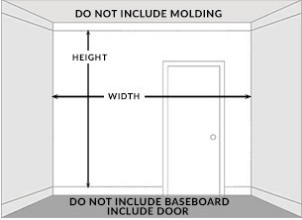• Measure the height (in feet or cm) and the width (in inches or cm) of each wall
• Do not include the crown molding
• Do not worry about doors or windows - it is unlikely that panels will align perfectly with these features
• For walls of varied heights, order rolls for the tallest wall to ensure the design repeat aligns properly across all panels

## Determine How Much Wallpaper to Order Using Our Wallpaper Calculator

Once you have your measurements, you can use this wallpaper calculator to estimate how many rolls to order. However, we highly recommend using design specific calculator found on every wallpaper product page because it applies the vertical design repeat and pattern repeat in its calculations.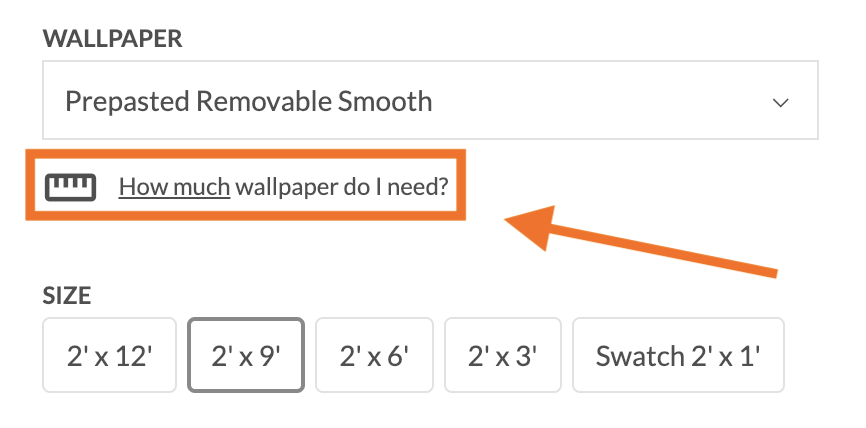1. From the design page, select your preferred WALLPAPER type
2. Link to the calculator through How much wallpaper do I need?
3. Enter the height and width from your measurements - depending upon your saved preferences - for each individual wall (see image below)
4. Click Calculate to see how many rolls, and what roll length, to order
5. Click Add to Cart, or Calculate twice, buy once and calculate manually as well!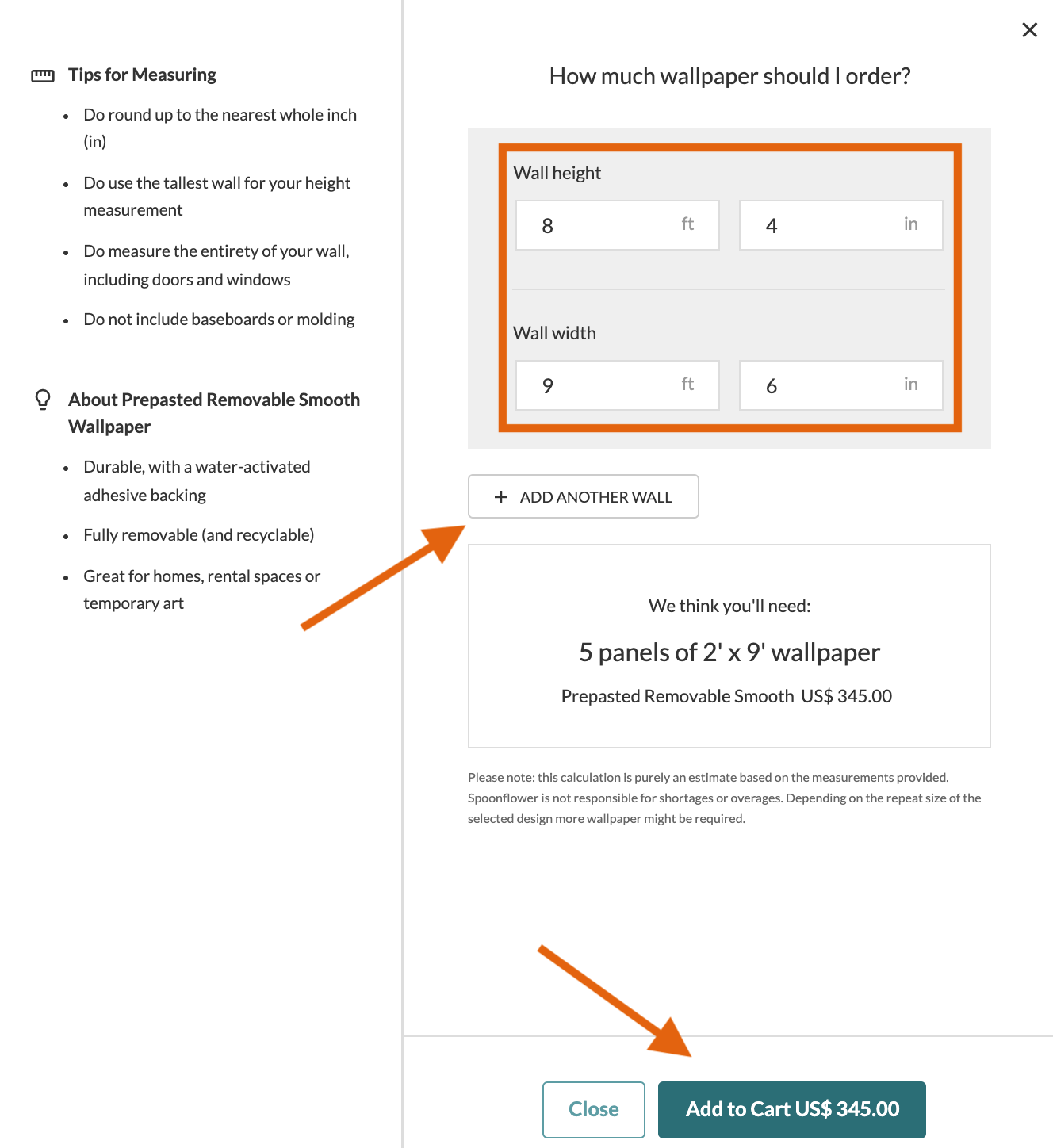## Ordering Wallpaper from the Marketplace

Review the example below for reference when ordering test swatches or rolls of wallpaper. SIZE will vary depending upon the WALLPAPER type you select.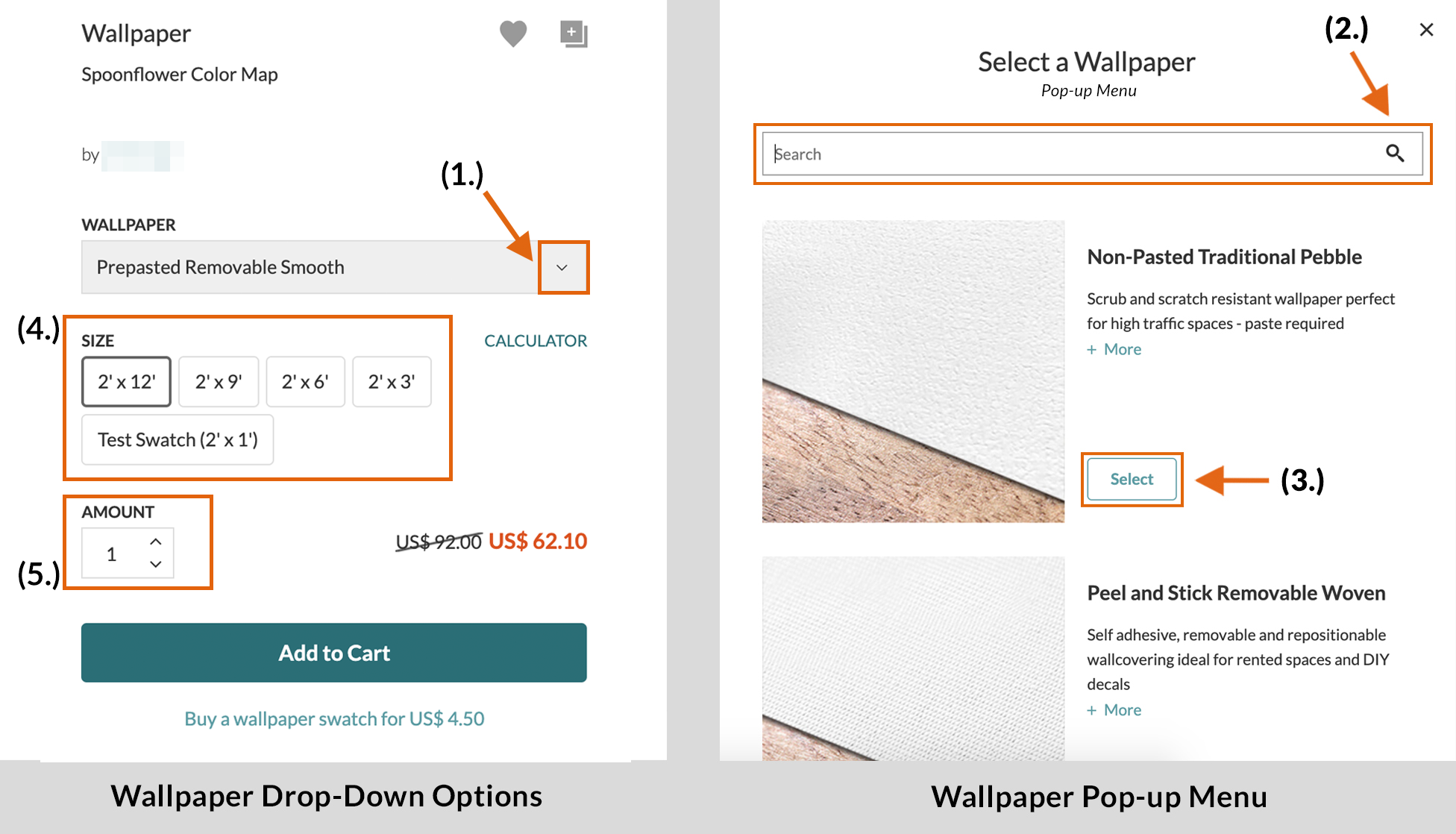### Ordering a Test Swatch of Wallpaper

We always recommend ordering a sample to ensure the wallpaper type, color and scale meet your expectations. It is also wise to install this Test Swatch, waiting a full 24 hours after installation to be certain it adheres correctly, before placing your larger order.

To order a Test Swatch

2. Search by name or scroll down to select from our four paper types
3. Click Select to chose that paper type
4. Highlight Test Swatch button from SIZE
5. Enter the AMOUNT - number of Test Swatches - you want to order

### Ordering Rolls

Follow steps (1.), (2.) and (3.) from above. Select (4.) the preferred roll SIZE and (5.) the number or rolls - AMOUNT based upon your calculations.

## Important Information about Print Lots (Dye Lots)

Customers often write in asking to order more paper from a certain dye-lot, or what we call a print-lot. Since Spoonflower is print-on-demand company, each individual order is actually its very own print-lot. While large color shifts between orders should never be an issue, slight variations may exist across two orders placed at different times. Therefore, it is important to order enough digitally printed paper to complete your entire installation all at once.

## Calculate How Many Rolls to Order Manually

If you want to check the math and calculate how much paper to order manually, follow one of the following two formulas below. Which formula depends upon the type of wallpaper - Smooth, Peel and Stick, or Pebble - that you are planning to purchase.

For Prepasted Removable Smooth and Peel and Stick Wallpaper:

We print these two popular Do-it-Yourself papers in selected whole-foot (or round centimeter) increments. It is good practice to order slightly longer rolls in order to ensure that any vagaries of architecture or slight differences in height along the wall can be compensated for during installation.

Imperial Measurement (inches and feet)

1. Round the height of your wall to the nearest roll length (3, 6, 9, 12 feet).

Example: If your wall measures just under 8 feet, not including the crown moulding, round up to 9 feet.(Note: If you are ordering for multiple walls in the same room, use the tallest wall height so that all the rolls are the same length.)

2. Calculate how many rolls you need

Take the full width of your wall (in inches) and divide it by 24”. If you will be installing on multiple walls in the same room that touch each other, add up the widths of each wall and divide that number by 24”. If the walls are not continuous, simply use this formula to determine the number of rolls necessary for each wall individually.

3. Round this answer up to the full number and order that many rolls of wallpaper.

Metric Measurement

1. Round the height of your wall to the nearest roll length (100 cm, 260 cm, 310 cm, or 400 cm).

Example: If your wall measures just under 220 cm, round up to 260 cm.(Note: If you are ordering for multiple walls in the same room, use the tallest wall height so that all the rolls are the same length.)

2. Calculate how many rolls you need

Take the full width of your wall (in centimeters) and divide it by 61 cm. If you will be installing on multiple walls in the same room that touch each other, add up the widths of each wall and divide that number by 61 cm.

3. Round this answer up to the full number and order that many rolls of wallpaper.

One roll of Traditional Pebble measures 27 feet (Imperial) or 10 meters (Metric), to covers approximately 36-40 square feet (6 square meters) once trim and pattern repeat are factored in. This may vary depending on wall height, design scale, and other factors.

Since every order is print-on-demand and color can vary between print runs, ensure you order enough rolls by giving yourself a little wiggle room in calculations. Rounding up, when directed, is important.

Imperial Measurement (inches and feet)

1. Take the measurements of the wall(s) you will be covering.

For our example we have two walls. One is 142" W x 108” H, and the other: 76" W x 108” H

2. Determine the number of panels you'll need.

• Add the widths of all the walls you are planning to cover
• Divide that number by the width of the roll of paper, 24" (2')
• Round this number to the next whole number. This is the number of panels.

Example: 142” + 76” = 218”; 218” divided by 24" = 9.08 panels; rounded: 10

3. Find the vertical repeat of your design.

You will find the vertical repeat on the design preview page of every wallpaper design. Scroll down from the top of the page to the “WALLPAPER DETAILS” box on the left and Click “+ More”. The first line of information is the vertical repeat measurement. (see screenshot below)

Example: 10.3" vertical repeat of the design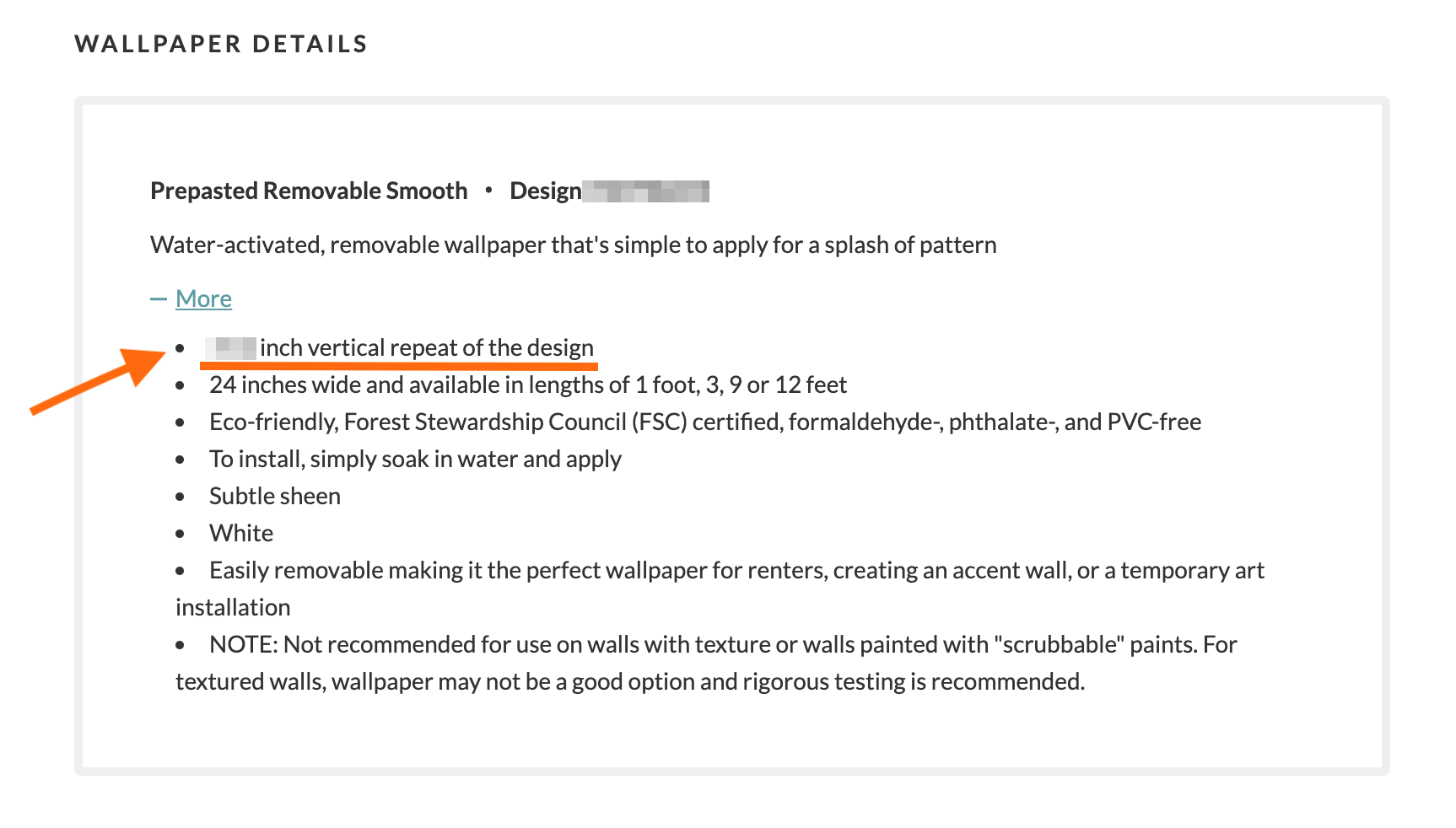4. Find the number of vertical repeats on one roll of Pebble.

Each roll of Pebble Wallpaper is 324" (27') long. Divide 324" by the vertical repeat from Step 3.

Example: 324” divided by 10.3” = 31.5 repeats per roll

5. Find the number of vertical repeats for one panel on the wall.

• Add 5" to the height of your installation wall (Step 1) for the height of each panel
• Divide this panel height by the vertical repeat of the design
• Round this number up to the next whole number. This is the repeats per panel

Example: 108” + 5” = 113”; 113” divided by 10.3” = 10.97; rounded: 11

6. Find the number of panels one roll of Pebble will cover.

Divide the number of vertical repeats per roll (Step 4) by the number of vertical repeats per panel (Step 5). Do NOT round.

Example: 31.5 divided by 11 = 2.9 panels per 27' roll

7. Calculate the number of rolls you will need to order.

Divide the total number of panels needed (Step 2) by the number of panels covered by one roll of Pebble (Step 6) and round up to the nearest whole number.

Example: 10 divided by 2.9 = 3.4 rolls. Rounded: 4 rolls

8. Order 4 rolls of this paper

Metric Measurement

1. Take the measurements of the wall(s) you will be covering.

For this example we will be installing wallpaper on two walls. One is 360 cm W x 270 cm H, and the other: 220 cm W x 270 cm H

2. Determine the number of panels you'll need.

• Add the widths of all the walls you are planning to cover
• Divide that number by the width of the roll of paper, 61 cm
• Round this number to the next whole number. This is the number of panels

Example: 360 cm + 220 cm = 580 cm; 580 cm divided by 61 cm = 9.51 panels; rounded: 10

3. Find the vertical repeat of your design.

You will find the vertical repeat on the design preview page of every wallpaper design. Scroll down from the top of the page to the “WALLPAPER DETAILS” box on the left and Click “+ More”. The first line of information is the vertical repeat measurement. (see screenshot below)

Example: 61.21 cm vertical repeat of the design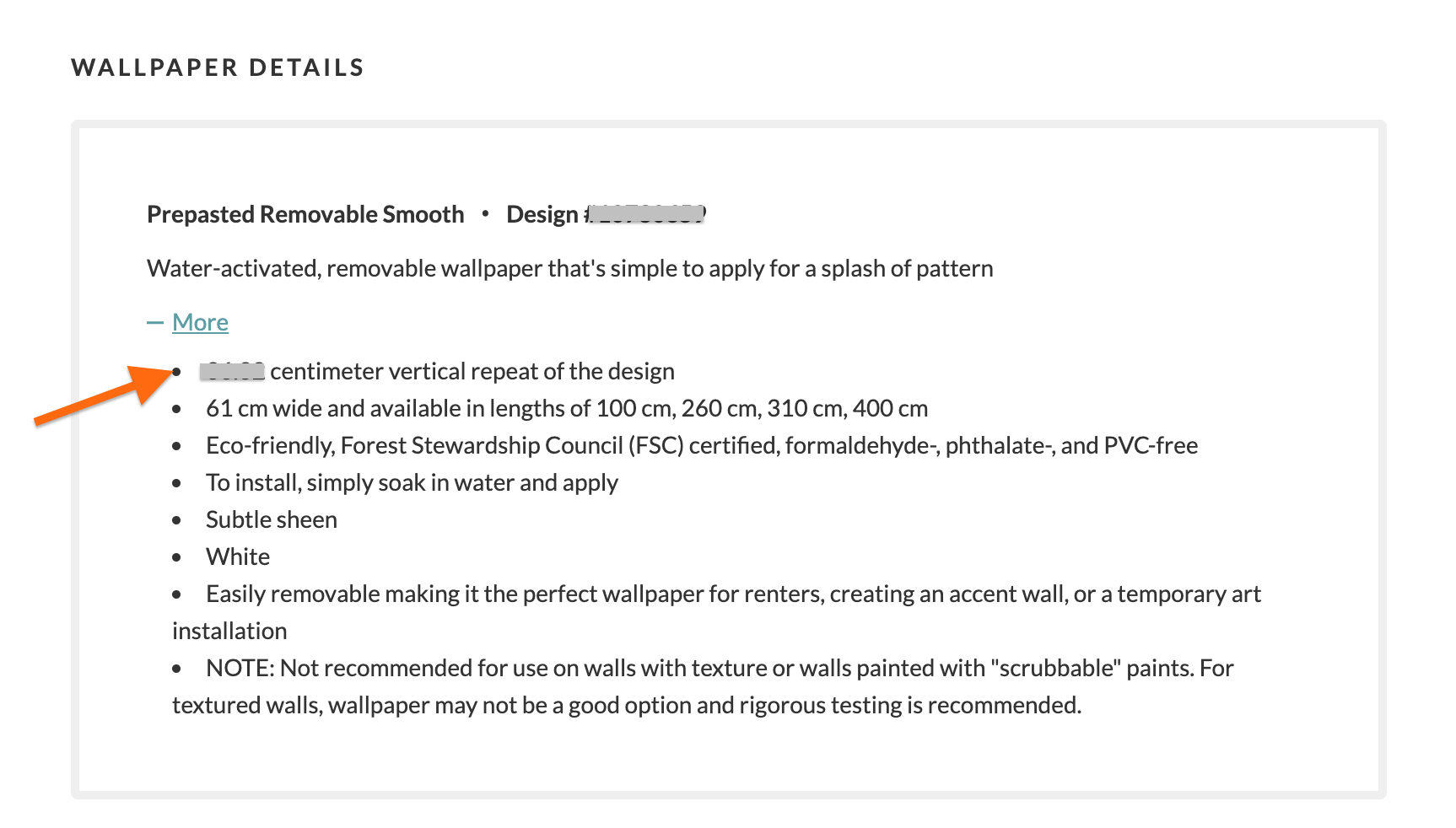4. Find the number of vertical repeats on one roll of Pebble.

Each roll of Pebble Wallpaper is 1000 cm (10 m) long. Divide 1000 cm by the vertical repeat from Step 3.

Example: 1000 cm divided by 61.21 cm = 16.34 repeats per roll

5. Find the number of vertical repeats for one panel on the wall.

• Add 12 cm to the height of your installation wall (from Step 1) to determine the height of each panel
• Divide this panel height by the vertical repeat of the design
• Round this number to the next whole number. This is the repeats per panel

Example: 270 cm + 12 cm = 282 cm; 282 cm divided by 61.21 cm = 4.61; rounded: 5

6. Find the number of panels one roll of Pebble will cover.

Divide the number of vertical repeats per roll (from Step 4) by the number of vertical repeats per panel (from Step 5). Do NOT round.

Example: 16.34 divided by 5 = 3.27 panels per 10 meter roll

7. Calculate the number of rolls you will need to order.

Divide the total number of panels needed (from Step 2) by the number of panels covered by one roll of Pebble (from Step 6) and round up to the nearest whole number.

Example: 10 divided by 3.27 = 3.06 rolls. Rounded: 4 rolls

8. Order 4 rolls of this paper